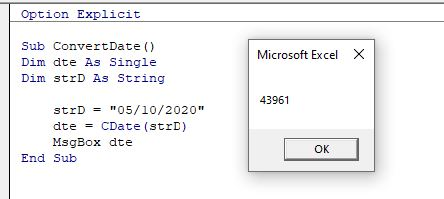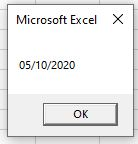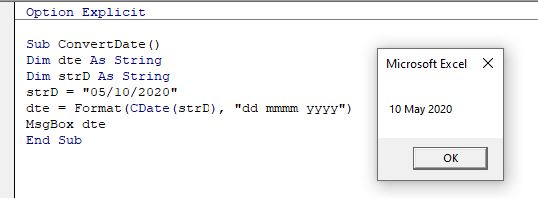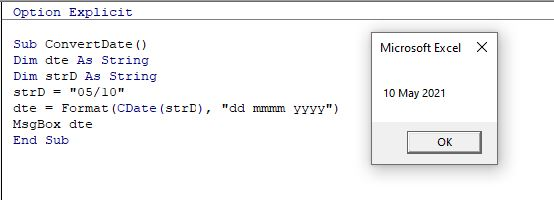# VBA CDate Function – Convert String to Date

This short tutorial will demonstrate how to convert a String to a Date in VBA.

Dates in Excel are stored as numbers, and then formatted to show as a date. Day 1 in the world of Excel was the 1st January 1900 (Windows default) or 1st January 1904 (Macintosh default) – which means that the 5th August 2021 is day 44413 since the 1st January 1900. To convert a string to a date in Excel, we first need to convert the string to a number, and then convert that number to the date.

## CDate Function

We can use a function called CDate in VBA to convert a string to a date.

``````Sub ConvertDate()
Dim dte As Single
Dim strD As String
strD = "05/10/2020"
dte = CDate(strD)
MsgBox dte
End Sub``````

As we have declared a numeric variable (dte as Single), the msgbox will return the number that refers to the date entered.It is important that we enter the year using all 4 of the year digits (ie 2020 and not just 20), otherwise the number returned might not be as expected.   Excel does not interpret the year part of the date – this is controlled by the Control Panel of our PC.

However, if we declare the variables as a date variable, the message box will return the number converted to a date.

``````Sub ConvertDate()
Dim dte As Date
Dim strD As String
strD = "05/10/2020"
dte = CDate(strD)
MsgBox dte
End Sub``````We can take this one step further and format the date to the type of date format that we would like to see.

``````Sub ConvertDate()
Dim dte As String
Dim strD As String
strD = "05/10/2020"
dte = Format(CDate(strD), "dd mmmm yyyy")
MsgBox dte
End Sub``````

In this example, we are converting the string to a date, and then back to a string again!If we were to omit the year entirely, Excel assumes the current year.Stop searching for VBA code online. Learn more about AutoMacro - A VBA Code Builder that allows beginners to code procedures from scratch with minimal coding knowledge and with many time-saving features for all users!